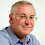# LISP Tutorial AI Artificial Intelligence LISP Programming Basic Introduction

LISP Tutorial On-line,  AI Artificial Intelligence LISP Programming Basic Introduction with examples
In AI history IPL (Information Processing Language) is often considered to be first language in which first AI program, The Logic Theorist, was written, (by Allen Newell, J. C. Shaw, and Herbert Simon). Later on LISP became the most popular language for the development of AI programs.  LISP is an acronym for “LISt Processor”. Symbolic processing languages are the languages specifically designed for the manipulation of symbols and writing AI programs. These often also termed as “list processing” languages. LISP was developed in 1958 at MIT (Massachusetts Institute of Technology), by John McCarthy. Some important features of lisp are given below:

Ø  Procedural/Functional
Ø  Symbolic manipulation (each language for specific task)
Ø  Syntax flexible (macros)
Ø  No distinction between data and code
Ø  Object Oriented Programming (CLOS)
Ø  Garbage Collection
Ø  S-expression  (atom, list)
Ø  List (small parenthesis) is basis of program, List is main data structure
Ø  False = nil = ( ) = empty list
Ø  Comments  ( ;  comment  #|  multilane comment |#)
Ø  Case sensitivity
Ø  Quote, set, Setq, functions
Ø  Predicate functions (null,  atom, listp, consp, oddp etc)
Ø  Conditionals (if , cond , or , and , not)

1.      SYNTAX
Ø  Prefix syntax
Ø  Simple Function Calls ( + , - , / , * , ) , embedded function calls

2.      EVALUATION RULE
Ø  A Number, string or special symbol ( T/ nil) is evaluated to self.
Ø   A Symbol is evaluated to its value.
Ø  For List ,  first element of the list should a function name, arguments (remaining elements of the list) are evaluated first (only once), then the function is executed.
Ø  Quote is a special function which stops the evaluation of its argument.
Ø  Setq also did not allow the evaluation of its first argument (which should be a symbol), but its second argument is evaluated then it assign the value of second argument to the first symbol.

3.      LIST ACCESSING (car, first, cdr, rest, second, third, nth etc.)
> (car ‘( a  b  c))
A
> (cdr ‘( a  b  c))
( B    C)

> (second ‘( a  b  c))
B
> (tenth  ‘( a  b  c))
nil

> (car ‘( (a  b  c))      )
(A B  C)

> (cdr ‘( (a  b  c) )    )
nil

> (nth  1 ‘( a  b  c )    )
B

> (last   ‘( a  b  c)        )
(C)

4.      LIST MANIPULATING (length, member, equal, position, subseq etc)
> (length   ‘( a  b  c)        )
3
> (member  ‘b   ‘( a  b  c)        )
(B C)
> (position  ‘b   ‘( a  b  c)        )
1

> (subseq    ‘( a  b  c   d    e    f     g)    2    5        )
(C   D    E)

5.      LIST CREATING (Cons, List, Append)
> (cons ‘K nil)
( K )

> (cons ‘(A   B)  (cons ‘C nil)  )
((A   B)   C)

> (cons ‘A (cons ‘b (cons ‘c nil)))
(A   B   C)

> (list ‘A  ‘B  ‘C )
(A   B   C)

> (list ‘A   nil    ( )  100  ‘(x  y  z)   ‘hello  )
(A   nil    nil  100  (x  y  z)   hello)

> (append    ‘(A)   ‘(B)    ‘(C)  ‘(D)   )
(A   B   C  D)

> (append    ‘((A)  B)    ‘(C)  ‘(((D)))   )
((A)   B   C  ((D)) )
##### 8.   PREDICATES  (numberp, listp, atom, symbolp, zerop, oddp, evenp, stringp, equal)

> (numberp  10)
T

> (symbpolp  ‘abc)
T

> (atom  10)
T

> (listp  10)
nil

> (listp  ‘( 1 0) )
T

> (null  ( ))
T

> (null  nil)
T
> (stringp  ‘abc)
nil

> (stringp  “abc”)
T

> (equal  ‘abc   ‘ABC)
T

> (equal  ‘(x y z)   ‘(x  y  z) )
T• Title : LISP Tutorial AI Artificial Intelligence LISP Programming Basic Introduction
• Posted by :
• Date : 10:33
• Labels :

1.